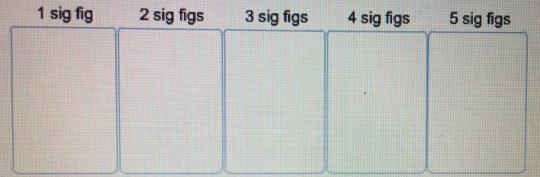# Problem: Identify the number of significant figures (sig figs) in each of these values.Note: If any part of this question is answered incorrectly, a single red X will appear702.50, 100, 6700, 7.000, 0.006, 0.030, 11020

###### FREE Expert Solution

In this problem, we are going to practice counting significant figures.

We'll use the following rules:

Rule 1. Zeros between digits are always significant.

Rule 2. Zeros to the left are never significant.

86% (290 ratings)###### Problem Details

Identify the number of significant figures (sig figs) in each of these values.

Note: If any part of this question is answered incorrectly, a single red X will appear702.50, 100, 6700, 7.000, 0.006, 0.030, 11020

Frequently Asked Questions

What scientific concept do you need to know in order to solve this problem?

Our tutors have indicated that to solve this problem you will need to apply the Counting Significant Figures concept. You can view video lessons to learn Counting Significant Figures. Or if you need more Counting Significant Figures practice, you can also practice Counting Significant Figures practice problems.

What professor is this problem relevant for?

Based on our data, we think this problem is relevant for Professor Dhalla's class at UCF.